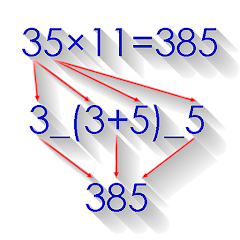# Math Tricks

4.5
410K reviews
10M+Everyone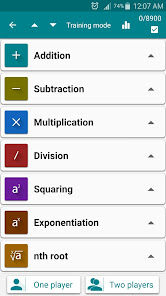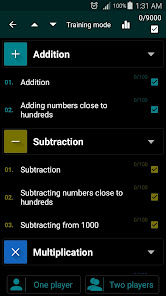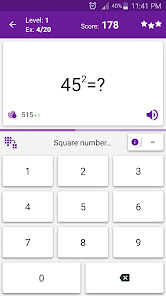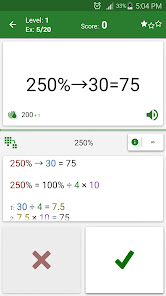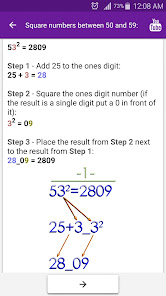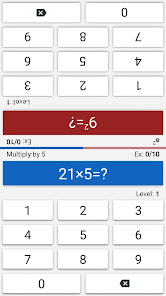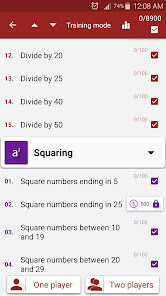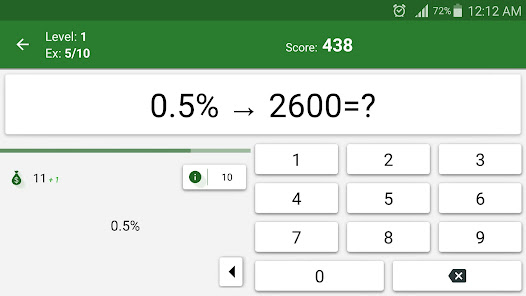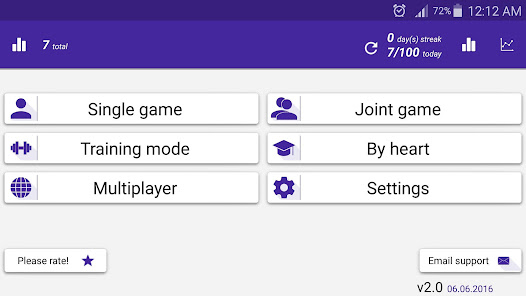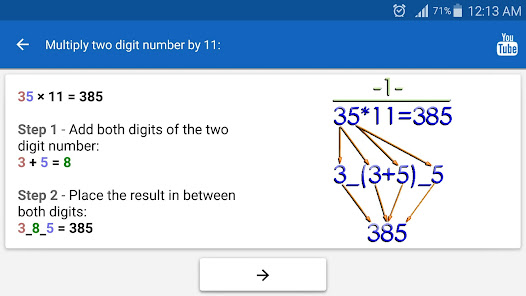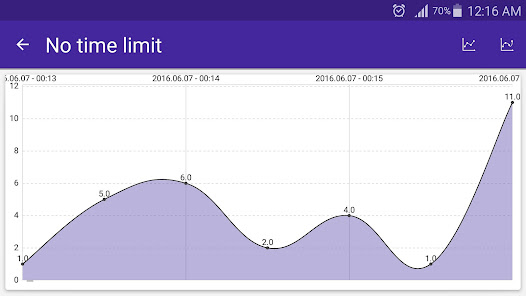This program is designed for those who want to learn interesting mathematical tricks to speed up the calculating. These tricks will help solve part of the mathematical problems and tasks much faster than classical. Will also be helpful to those who want to hone basics such as the multiplication table.

When you learn these mathematical tricks, you'll be able to show off your skills to friends and prove to them that you have a talent for mathematics. New skills you can use in the store, at school, at college, at work - wherever thanks to quick calculation skills can save a lot of precious time.

Fun Math Tricks:
2. Subtraction
3. Multiplication table
4. Division
5. Multiply two digit number by 11
6. Square numbers ending in 5
7. Multiply by 5
8. Multiply by 9
9. Multiply by 4
10. Dividing by 5
11. Subtracting from 1000
12. Tough multiplication
13. Power of two
14. Adding numbers close to hundreds
15. Subtracting numbers close to hundreds
16. Multiply numbers between 11 and 19
17. Square numbers between 11 and 99
18. Multiply two digit numbers having same tens digit and ones digits add up to ten
19. Square numbers between 50 and 59
20. Square numbers between 40 and 49
21. Multiply two digit numbers editing in 1
22. Percent
23. Square numbers between 100 and 109
...
84. n^9

Training mode:
- 15 levels for every trick
- timer 15 seconds
Rating stars:
- score > 000 - ★☆☆
- score > 350 - ★★☆
- score > 650 - ★★★

This free version of Math Tricks contains third party advertisements.

The app uses the following APIs and libraries:
* MPAndroidChart - created by Philipp Jahoda
* ColorPIcker - created by Piotr Adamus
Updated on
Oct 25, 2021

## Data safety

Developers can show information here about how their app collects and uses your data. Learn more about data safetyNo information available
4.5
410K reviews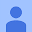November 24, 2018
It was very detailed. I appreciate that, unlike some apps or websites that give math shortcuts, it not only gives the trick, it explains the reasoning behind it, and gives many exercises afterwards to ensure that you understand. The math tricks are effective and save a lot of time that would otherwise be spent writing out the entire problem and using the traditional way. My only concern is that there are a little too many ads. Ads put aside, it is a very high quality app that I would recommend to those having trouble with math, those studying for a timed test, or simply those who want to save tons of time on math.
380 people found this review helpful
Did you find this helpful?December 29, 2019
Great app, useful for so many reasons, but for the timers! Argh! I couldn't figure out how to turn them off (I'm not even certain you are able to...?) and they added needless stress. There are other apps that are comparable (withOUT the short timer) though not as comprehensive, so if you're also stressed by timers, go elsewhere. App developers, is it possible to take the timerrs off? Am I missing something?
44 people found this review helpful
Did you find this helpful?